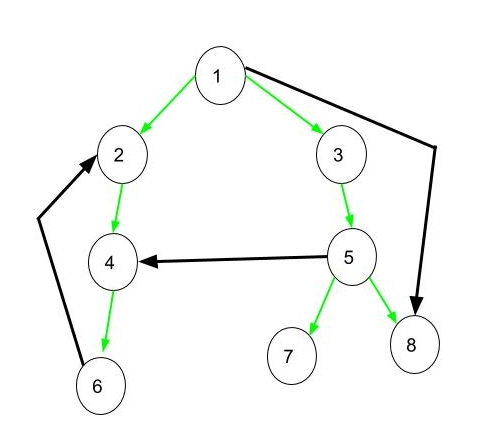Open in App
Not now

# Tree, Back, Edge and Cross Edges in DFS of Graph

• Difficulty Level : Easy
• Last Updated : 02 Sep, 2022

Consider a directed graph given in below, DFS of the below graph is 1 2 4 6 3 5 7 8. In below diagram if DFS is applied on this graph a tree is obtained which is connected using green edges.• Tree Edge: It is an edge which is present in the tree obtained after applying DFS on the graph. All the Green edges are tree edges.
• Forward Edge: It is an edge (u, v) such that v is a descendant but not part of the DFS tree. An edge from 1 to 8 is a forward edge.
• Back edge: It is an edge (u, v) such that v is the ancestor of node u but is not part of the DFS tree. Edge from 6 to 2 is a back edge. Presence of back edge indicates a cycle in directed graph
• Cross Edge: It is an edge that connects two nodes such that they do not have any ancestor and a descendant relationship between them. The edge from node 5 to 4 is a cross edge.

Time Complexity(DFS):

Since all the nodes and vertices are visited, the average time complexity for DFS on a graph is O(V + E), where V is the number of vertices and E is the number of edges. In case of DFS on a tree, the time complexity is O(V), where V is the number of nodes.

Algorithm(DFS):

• Pick any node. If it is unvisited, mark it as visited and recur on all its adjacent nodes.
• Repeat until all the nodes are visited, or the node to be searched is found.

Example: Implement DFS using an adjacency list take a directed graph of size n=10, and randomly select the number of edges in the graph varying from 9 to 45. Identify each edge as the forwarding edge, tree edge, back edge, and cross edge.

## Python3

 `# code``import` `random`  `class` `Graph:``    ``# instance variables``    ``def` `__init__(``self``, v):``        ``# v is the number of nodes/vertices``        ``self``.time ``=` `0``        ``self``.traversal_array ``=` `[]``        ``self``.v ``=` `v``        ``# e is the number of edge (randomly chosen between 9 to 45)``        ``self``.e ``=` `random.randint(``9``, ``45``)``        ``# adj. list for graph``        ``self``.graph_list ``=` `[[] ``for` `_ ``in` `range``(v)]``        ``# adj. matrix for graph``        ``self``.graph_matrix ``=` `[[``0` `for` `_ ``in` `range``(v)] ``for` `_ ``in` `range``(v)]` `    ``# function to create random graph``    ``def` `create_random_graph(``self``):``        ``# add edges upto e``        ``for` `i ``in` `range``(``self``.e):``            ``# choose src and dest of each edge randomly``            ``src ``=` `random.randrange(``0``, ``self``.v)``            ``dest ``=` `random.randrange(``0``, ``self``.v)``            ``# re-choose if src and dest are same or src and dest already has an edge``            ``while` `src ``=``=` `dest ``and` `self``.graph_matrix[src][dest] ``=``=` `1``:``                ``src ``=` `random.randrange(``0``, ``self``.v)``                ``dest ``=` `random.randrange(``0``, ``self``.v)``            ``# add the edge to graph``            ``self``.graph_list[src].append(dest)``            ``self``.graph_matrix[src][dest] ``=` `1` `    ``# function to print adj list``    ``def` `print_graph_list(``self``):``        ``print``(``"Adjacency List Representation:"``)``        ``for` `i ``in` `range``(``self``.v):``            ``print``(i, ``"-->"``, ``*``self``.graph_list[i])``        ``print``()` `    ``# function to print adj matrix``    ``def` `print_graph_matrix(``self``):``        ``print``(``"Adjacency Matrix Representation:"``)``        ``for` `i ``in` `self``.graph_matrix:``            ``print``(i)``        ``print``()` `    ``# function the get number of edges``    ``def` `number_of_edges(``self``):``        ``return` `self``.e` `    ``# function for dfs``    ``def` `dfs(``self``):``        ``self``.visited ``=` `[``False``]``*``self``.v``        ``self``.start_time ``=` `[``0``]``*``self``.v``        ``self``.end_time ``=` `[``0``]``*``self``.v` `        ``for` `node ``in` `range``(``self``.v):``            ``if` `not` `self``.visited[node]:``                ``self``.traverse_dfs(node)``        ``print``()``        ``print``(``"DFS Traversal: "``, ``self``.traversal_array)``        ``print``()` `    ``def` `traverse_dfs(``self``, node):``        ``# mark the node visited``        ``self``.visited[node] ``=` `True``        ``# add the node to traversal``        ``self``.traversal_array.append(node)``        ``# get the starting time``        ``self``.start_time[node] ``=` `self``.time``        ``# increment the time by 1``        ``self``.time ``+``=` `1``        ``# traverse through the neighbours``        ``for` `neighbour ``in` `self``.graph_list[node]:``            ``# if a node is not visited``            ``if` `not` `self``.visited[neighbour]:``                ``# marks the edge as tree edge``                ``print``(``'Tree Edge:'``, ``str``(node)``+``'-->'``+``str``(neighbour))``                ``# dfs from that node``                ``self``.traverse_dfs(neighbour)``            ``else``:``                ``# when the parent node is traversed after the neighbour node``                ``if` `self``.start_time[node] > ``self``.start_time[neighbour] ``and` `self``.end_time[node] < ``self``.end_time[neighbour]:``                    ``print``(``'Back Edge:'``, ``str``(node)``+``'-->'``+``str``(neighbour))``                ``# when the neighbour node is a descendant but not a part of tree``                ``elif` `self``.start_time[node] < ``self``.start_time[neighbour] ``and` `self``.end_time[node] > ``self``.end_time[neighbour]:``                    ``print``(``'Forward Edge:'``, ``str``(node)``+``'-->'``+``str``(neighbour))``                ``# when parent and neighbour node do not have any ancestor and a descendant relationship between them``                ``elif` `self``.start_time[node] > ``self``.start_time[neighbour] ``and` `self``.end_time[node] > ``self``.end_time[neighbour]:``                    ``print``(``'Cross Edge:'``, ``str``(node)``+``'-->'``+``str``(neighbour))``            ``self``.end_time[node] ``=` `self``.time``            ``self``.time ``+``=` `1`  `if` `__name__ ``=``=` `"__main__"``:``    ``n ``=` `10``    ``g ``=` `Graph(n)``    ``g.create_random_graph()``    ``g.print_graph_list()``    ``g.print_graph_matrix()``    ``g.dfs()`

Output

```Adjacency List Representation:
0 --> 5
1 --> 3 7
2 --> 4 3 8 9
3 --> 3
4 --> 0
5 --> 2 0
6 --> 0
7 --> 7 4 3
8 --> 8 9
9 --> 9

[0, 0, 0, 0, 0, 1, 0, 0, 0, 0]
[0, 0, 0, 1, 0, 0, 0, 1, 0, 0]
[0, 0, 0, 1, 1, 0, 0, 0, 1, 1]
[0, 0, 0, 1, 0, 0, 0, 0, 0, 0]
[1, 0, 0, 0, 0, 0, 0, 0, 0, 0]
[1, 0, 1, 0, 0, 0, 0, 0, 0, 0]
[1, 0, 0, 0, 0, 0, 0, 0, 0, 0]
[0, 0, 0, 1, 1, 0, 0, 1, 0, 0]
[0, 0, 0, 0, 0, 0, 0, 0, 1, 1]
[0, 0, 0, 0, 0, 0, 0, 0, 0, 1]

Tree Edge: 0-->5
Tree Edge: 5-->2
Tree Edge: 2-->4
Tree Edge: 2-->3
Tree Edge: 2-->8
Tree Edge: 8-->9
Forward Edge: 2-->9
Cross Edge: 5-->0
Back Edge: 1-->3
Tree Edge: 1-->7
Cross Edge: 7-->4
Cross Edge: 7-->3
Back Edge: 6-->0

DFS Traversal:  [0, 5, 2, 4, 3, 8, 9, 1, 7, 6]```

My Personal Notes arrow_drop_up